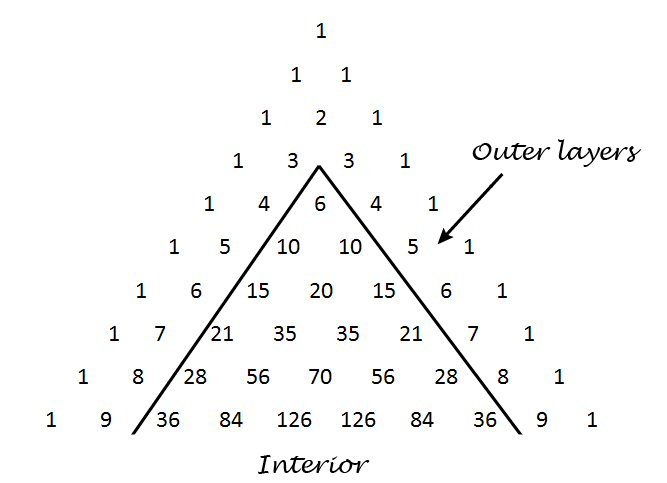# Distribution of Numbers in Pascal's Triangle

### This post uses Python code to explore a sentence from the book "Single Digits": Any number in Pascal’s triangle that is not in the outer two layers will appear at least three times, usually four.

· Big Data Zone · Analysis
Save
3.73K Views

this post explores a sentence from the book single digits :

any number in pascal’s triangle that is not in the outer two layers will appear at least three times, usually four.

pascal’s triangle contains the binomial coefficients c( n , r ) where n ranges over non-negative numbers and r ranges from 0 to n . the outer layers are the elements with r equal to 0, 1, n -1, and n .we’ll write some python code to explore how often the numbers up to 1,000,000 appear. how many rows of pascal’s triangle should we compute? the smallest number in row n is c( n , 2). now 1,000,000 is between c(1414, 2) and c(1415, 2) so we need row 1414. this means we need n = 1415 below because the row numbers start with 0.

i’d like to use a numpy array for storing pascal’s triangle. in the process of writing this code, i realized that a numpy array with ``` dtype int ``` doesn’t contain python’s arbitrary-sized integers. this makes sense because numpy is designed for efficient computation, and using a numpy array to contain huge integers is unnatural. but i’d like to do it anyway, and the to make it happen is to set ``` dtype ``` to ``` object ``` .

``````    import numpy as np
from collections import counter

n = 1415 # number of rows of pascal's triangle to compute

pascal = np.zeros((n, n), dtype=object)
pascal[0, 0] = 1
pascal[1,0] = pascal[1,1] = 1

for n in range(2, n):
for r in range(0, n+1):
pascal[n, r] = pascal[n-1, r-1] + pascal[n-1, r]

c = counter()
for n in range(4, n):
for r in range(2, n-1):
p = pascal[n, r]
if p <= 1000000:
c[p] += 1
``````

when we run this code, we find that our counter contains 1732 elements. that is, of the numbers up to one million, only 1732 of them appear inside pascal’s triangle when we disallow the outer two layers. (the second layer contains consecutive integers, so every positive integer appears in pascal’s triangle. but most integers only appear in the second layer.)

when single digits speaks of “any number in pascal’s triangle that is not in the outer two layers” this cannot refer to numbers that are not in the outer two layers because every natural number appears in the outer two layers. also, when it says the number “will appear at least three times, usually four” it is referring to the entire triangle, i.e. including the outer two layers. so another way to state the sentence quoted above is as follows.

define the interior of pascal’s triangle to be the triangle excluding the outer two layers. every number n in the interior of pascal’s triangle appears twice more outside of the interior, namely as c( n , 1) and c( n , n -1). most often n appears at least twice in the interior as well.

this means that any number you find in the interior of pascal’s triangle, interior meaning not in the two outer layers, will appear at least three times in the full triangle, usually more.

here are the statistics from our code above regarding just the interior of pascal’s triangle:

• one number, 3003, appears six times.
• six numbers appear four times: 120, 210, 1540, 7140, 11628, and 24310.
• ten numbers appear only once: 6, 20, 70, 252, 924, 3432, 12870,  48620, 184756, and 705432.
• the large majority of numbers, 1715 out of 1732, appear twice.
Topics:
python, pascal, snippet

Published at DZone with permission of John Cook, DZone MVB.

Opinions expressed by DZone contributors are their own.